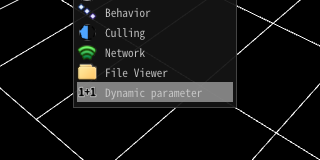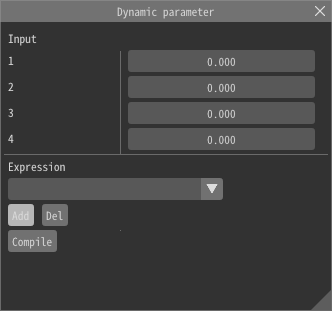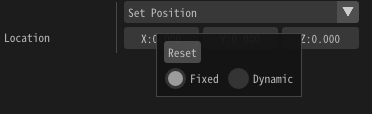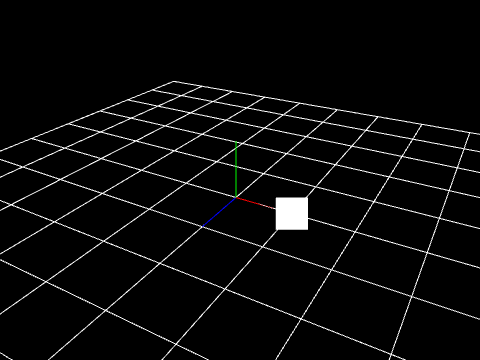# DynamicParameter¶

## Overview¶

DynamicParameter specifies a parameter to rewrite refresh (during game) effect parameters during effect playback. It controls parameters with simple code. Parameters can be given externally.

## How to use¶

### Basic¶

#### Panel¶

Open the dynamic parameter panel.You can add an expression with the add button. You can specify the name of the expression and the content of the expression.#### Write an expression¶

For example, to rewrite the first parameter to 2.0, write as follows.

```@O.x = 2.0
```

After that you press Compile. If no problem exists, OK is displayed. If a problem exists, the cause of error is displayed.

```@O.x = 2.0
```

#### Apply the expression¶

Right-click on the parameter to which it applies and select Dynamic. Then, a field for selecting the expression is displayed.Then, select the expression you entered earlier and the expression is applied.The parameter of the position is (0, 0, 0). But a particle is shown on 2 because the expression changes positions into 2### Functions of an expression¶

#### Change multiple parameters¶

x, y, z, w, each parameter can be changed. Entering the following changes the values ​​to 2, 3, 4, and 5, respectively. If the destination is a color, x, y, z, w correspond to RGBA.

```@O.x = 2.0
@O.y = 3.0
@O.z = 4.0
@O.w = 5.0
```

#### Four arithmetic operations¶

Expressions can use arithmetic operations. You can change the priority of the operation with ().

```@O.x = (2.0 + 3.0 * 2.0 / 3.0) - 2.0
```

#### Parameter¶

Parameters input from the external can be specified from the numeric field on the panel and runtime.

Parameter Description
@P.x @P.y @P.z @P.w Parameters before dynamic parameter is applied
@In0 @In1 @In2 @In3 Parameters input from the external
@GTime Current time(s)
@PTime A time since parent particle is generated (s)

#### Function¶

The following functions can be used.

Parameter Description
sin(x) Return the result of Sine. x is in radians.
cos(x) Return the result of Cosine. x is in radians.
rand() Return the random value
rand(x) Return the random value corresponding with x. If x is same, returned value is same
step(edge,x) Return 1.0 if x is larger than edge. Otherwise return 0.

#### Target¶

Dynamic parameter can applies to following parameters.

• Spawn Count

• Spawn Rate

• Initial Delay

• Time to live

• Position - Fixed, PVA, Easing

• Rotation - Fixed, PVA, Easing

• Scaling - Fixed, PVA, Easing

## Examples¶

### LOD¶

The number of generations can be changed externally.

```@O.x = @In0 * @P.x
```

### Laser¶

The length of the laser can be changed externally. The size of Z is controlled externally.

```@O.z = @In0 * @P.z
```

### Sine Wave¶

Move like a sine wave.

```@O.x = sin(@GTime)
@O.y = @GTime
```